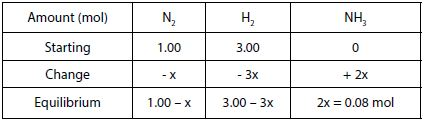Chemistry

# Haber’s Process

The Haber’s process for producing ammonia from N2 and H2 is an important industrial example of a reaction that does not go to completion.

It establishes an equilibrium state where all the three species are present.

Example: Suppose we place 1.00 mol of N2 and 3.00 mol of H2 in a reaction vessel at a temperature of 450 °C and pressure of 10.0 atm. The Haber’s reaction is:

N2 (g) + 3H2 (g) ↔ 2NH3 (g)

What is the composition of the equilibrium mixture if it contains 0.08 mol of NH3?

Solution: Using the information given, a table can be created in terms of “x”, the change in the number of moles of one reactant as shown in the table below:The equilibrium amount of NH3 is equal to 0.08 mol. Therefore, 2x = 0.08 mol and then x = 0.04 mol of NH3.

• Equilibrium amount of N2 = 1.00 – 0.04 = 0.96 mol of N2
• Equilibrium amount of H2 = 3.00 – (3 x 0.04) = 2.88 mol o H2
• Equilibrium amount of NH3 = 2 x = 0.08 mol of NH3

There is far more H2 and N2 present at equilibrium than NH3. This will not change, no matter how long the reaction is left. Being at equilibrium does not mean that the amount of reactants equals the amount of product.